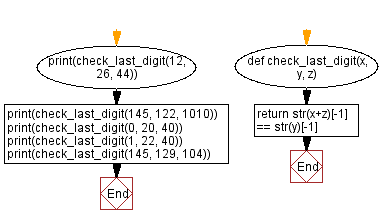﻿ Python: Sum of the last digit of first number and the last digit of second number equal to the last digit of third number - w3resource

# Python: Sum of the last digit of first number and the last digit of second number equal to the last digit of third number

## Python Basic - 1: Exercise-108 with Solution

Write a Python program that takes three integers and checks whether the sum of the last digit of the first number and the last digit of the second number equals the last digit of the third number.

Sample Solution:

Python Code:

``````def check_last_digit(x, y, z):
return str(x+z)[-1] == str(y)[-1]
print(check_last_digit(12, 26, 44))
print(check_last_digit(145, 122, 1010))
print(check_last_digit(0, 20, 40))
print(check_last_digit(1, 22, 40))
print(check_last_digit(145, 129, 104))
``````

Sample Output:

```True
False
True
False
True
```

Pictorial Presentation:Flowchart:Python Code Editor:

Have another way to solve this solution? Contribute your code (and comments) through Disqus.

What is the difficulty level of this exercise?

Test your Programming skills with w3resource's quiz.

﻿